Mechanics

# Vertical launch upwards

Vertical launch upwards is a one- dimensional movement that occurs when a body is thrown upwards and, therefore, with a non-zero initial velocity, undergoing a deceleration due to gravity . In this type of movement, the action of dissipative forces, such as the force of friction with the air, is disregarded. The vertical launch is one of the applications of uniformly varied rectilinear motion , being of great importance for the study of kinematics.

## Introduction to vertical launch

In the vertical upward launch, a particle moves in a straight line until it reaches a point of maximum height. At this point, the direction of motion is reversed and, then, this particle begins to describe a free fall motion .

During the ascent, the movement is slowed down by the action of gravity, which points towards the center of the Earth. Furthermore, the time it takes the particle to rise is equal to the time to fall , since the acceleration to which the particle is subjected remains the same.

To solve exercises on vertical launch, it is necessary to know the main time equations of the MRUV, as well as the Torricelli equation applied to vertical movement. In addition, it is essential to define an adequate frame of reference for the sign convention, therefore, as a suggestion, we suggest adopting the positive sign for the positive direction of the y (vertical) axis. In this way, the initial velocity will have a positive sign, while the acceleration due to gravity will have a negative sign. Such a reference facilitates the resolution of exercises on the vertical throw upwards.

Now that we know how to orient the frame properly, let’s get to know the main formulas used to study vertical motion.

## Vertical launch formulas

The main formulas used to study the vertical launch are the position and velocity time equations used in the MRUV. To use them, we make some adaptations, such as the symbols H F and H 0 , used to denote the final and initial heights, and the symbol g, to designate the acceleration due to gravity.

The velocity formula for the vertical launch is this: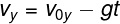y – final vertical velocity (m/s)

0y – initial vertical velocity (m/s)

g – acceleration due to gravity (m/s²)

t – instant of time (s)

Below you can see the formula for the position hourly function for the vertical launch: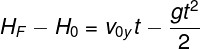Finally, we show in the following figure the Torricelli equation for the vertical launch. Watch: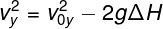## Solved exercises on vertical launch

Question 1 — Determine the maximum height reached by an object that is thrown upward with an initial speed of 20 m/s. Data: g = 10 m/s².

a) 40 m

b) 20 m

c) 2.0 m

d) 25 m

Resolution:

To solve the exercise, we use Torricelli’s equation: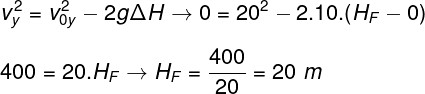Based on the result obtained, the correct alternative is the letter B .

Question 2 — An object is thrown upward in a region where gravity is 10 m/s². Assuming that it reached the highest point of its trajectory in 2 seconds, what was the speed at which this object was launched?

a) 20 m/s

b) 40 m/s

c) 10 m/s

d) 15 m/s

Resolution:

To solve this exercise, just remember that, at the point of maximum height, the speed of movement is zero. With this, it is possible to answer the question using the hourly speed function. Watch: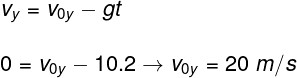Based on the calculation made above, we found that the launch speed of the object was 20 m/s. Thus, the correct alternative is the letter A .